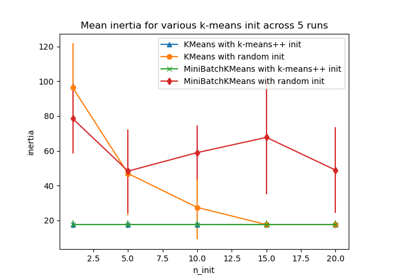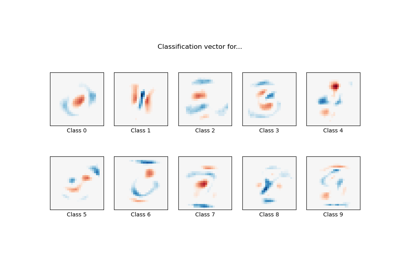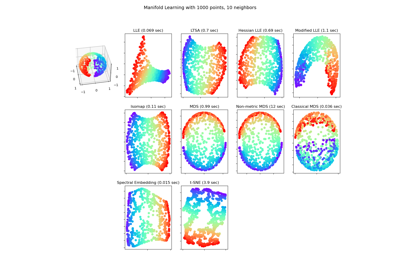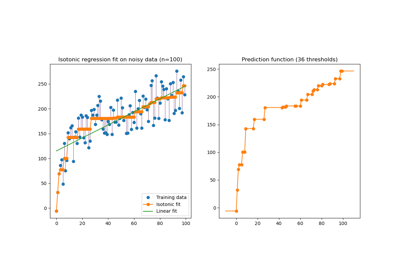# sklearn.utils.check_random_state¶

sklearn.utils.check_random_state(seed)[source]

Turn seed into a np.random.RandomState instance.

Parameters:
seedNone, int or instance of RandomState

If seed is None, return the RandomState singleton used by np.random. If seed is an int, return a new RandomState instance seeded with seed. If seed is already a RandomState instance, return it. Otherwise raise ValueError.

Returns:
numpy.random.RandomState

The random state object based on seed parameter.

## Examples using sklearn.utils.check_random_state¶Empirical evaluation of the impact of k-means initialization

Empirical evaluation of the impact of k-means initializationMNIST classification using multinomial logistic + L1

MNIST classification using multinomial logistic + L1Manifold Learning methods on a severed sphere

Manifold Learning methods on a severed sphereFace completion with a multi-output estimators

Face completion with a multi-output estimatorsIsotonic Regression

Isotonic Regression